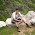## January 01, 2013

### Parallel to Serial Converter

Let's discuss the design of a Parallel to Serial Converter. Here's the crude problem statement.

You have 8 FFs, each working at a frequency of 10MHz and sending out a parallel data which effectively would be 8-bit wide. We intend to convert this data into a serial one, where 1 FF would output the data serially in order of first FF, then data of second FF and so on. Refer to the diagram below:
Such a parallel to serial converter might be useful in applications requiring Serial Communication which maybe interface to a microprocessor or a microcontroller; interface between your monitor and the CPU or any application which might involve serial processing of information.

Now let's proceed for the design. Note that:
• We have 8-bits of input data, and we want to output them one at a time. This suggests the use of a multiplexer. The size of this multiplexer would obviously be 8:1.
• Apart from the inputs, the multiplexer would have select lines (3 select lines for a 8:1 MUX) and we need to control these lines. Select lines need to be controlled in such a way that first the FF1 is selected, then FF2 and so on.
• Recall the functionality of a multiplexer. The first bit is selected when select lines would be {S2,S1,S0} = 000, Second bit when {S2,S1,S0} = 001. Hence we can use a binary counter which would count from 000 to 111 in a binary manner.
• What would be the frequency of this counter? Note that for every one cycle of input data, we need to 8 outputs corresponding to 8 input FFs. Hence the frequency of the counter would be:
Freq. = 8 X 10 MHz = 80 MHz
Here's the final design:

#### 5 comments:

1.If the figure 2 is the brown box in figure 1, the freq should be 10MHz, and the input data have to keep for 8 cycles for serialization. If 80Mhz, there must be a buffer and clock domain crossing circuit, or the output is unpredicable.

1.Hi Victor. Thanks for pointing it out. If these two clocks are asynchronous, clock domain cross circuit is indispensable. But, I meant these clocks to be synchronous. I should have rather make it more implicit, but I felt it was beyond the scope of this post.

On further thought, I am now curious to know why one out of the two methods would be more practical. If we consider the two clocks to be synchronous, one would need to generate a 80Meg clock signal from 10Meg signal. If asynchronous, clock domain crossing circuit would be needed, apart from the constraint that data needs to be retained for 8 clock cycles, which would mean lower throughout. Any idea how it's done?

2.Hi Naman,
The serializer ff should also be clocked at 80MHz I believe?

1.Hi Shri.

Yes, the serializer FF should also be clocked at 80 Meg, otherwise it would capture only 1 out of the 8 data bits. Thanks for pointing it out.

3.You should changed the last FFs clock source. As alternative, PISO (parallel in serial out shift register) can be used instead of this multiplexer + counter circuit. You can check this study,

http://ieeexplore.ieee.org/xpl/login.jsp?tp=&arnumber=6628276&url=http%3A%2F%2Fieeexplore.ieee.org%2Fxpls%2Fabs_all.jsp%3Farnumber%3D6628276